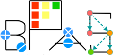Basic Polynomial Algebra Subprograms (BPAS)  v. 1.652
SmallPrimeField Class Reference

A prime field whose prime is 32 bits or less. More...

`#include <SmallPrimeField.hpp>`

Simplified semantic inheritance diagram for SmallPrimeField:Full inheritance diagram for SmallPrimeField:
[legend]

## Public Member Functions

SmallPrimeField (long long int _a)

SmallPrimeField (const SmallPrimeField &c)

SmallPrimeField (const Integer &c)

SmallPrimeField (const RationalNumber &c)

SmallPrimeField (const ComplexRationalNumber &c)

SmallPrimeField (const BigPrimeField &c)

SmallPrimeField (const GeneralizedFermatPrimeField &c)

SmallPrimeField (const DenseUnivariateIntegerPolynomial &c)

SmallPrimeField (const DenseUnivariateRationalPolynomial &c)

SmallPrimeField (const SparseUnivariatePolynomial< Integer > &c)

SmallPrimeField (const SparseUnivariatePolynomial< RationalNumber > &c)

SmallPrimeField (const SparseUnivariatePolynomial< ComplexRationalNumber > &c)

template<class Ring >
SmallPrimeField (const SparseUnivariatePolynomial< Ring > &c)

SmallPrimeFieldSPFpointer (SmallPrimeField *b)

SmallPrimeFieldSPFpointer (RationalNumber *a)

SmallPrimeFieldSPFpointer (BigPrimeField *a)

SmallPrimeFieldSPFpointer (GeneralizedFermatPrimeField *a)

long long int number () const

void whichprimefield ()

long long int Prime ()

SmallPrimeFieldoperator= (const SmallPrimeField &c)
Copy assignment.

SmallPrimeFieldoperator= (long long int k)

SmallPrimeField findPrimitiveRootOfUnity (long int n) const

bool isZero () const
Determine if *this ring element is zero, that is the additive identity. More...

void zero ()
Make *this ring element zero.

bool isOne () const
Determine if *this ring element is one, that is the multiplication identity. More...

void one ()
Make *this ring element one.

bool isNegativeOne ()

void negativeOne ()

int isConstant ()

SmallPrimeField unitCanonical (SmallPrimeField *u=NULL, SmallPrimeField *v=NULL) const
Obtain the unit normal (a.k.a canonical associate) of an element. More...

SmallPrimeField operator+ (const SmallPrimeField &c) const
Addition.

SmallPrimeField operator+ (const long long int &c) const

SmallPrimeField operator+ (const int &c) const

SmallPrimeField operator+= (const long long int &c)

SmallPrimeFieldoperator+= (const SmallPrimeField &c)
Addition assignment.

SmallPrimeField operator- (const SmallPrimeField &c) const
Subtraction.

SmallPrimeField operator- (const long long int &c) const

SmallPrimeField operator-= (const long long int &c)

SmallPrimeFieldoperator-= (const SmallPrimeField &c)
Subtraction assignment.

SmallPrimeField operator- () const
Negation.

SmallPrimeField operator* (const SmallPrimeField &c) const
Multiplication.

SmallPrimeField operator* (long long int c) const

SmallPrimeFieldoperator*= (const SmallPrimeField &c)
Multiplication assignment.

long long int * pinverse ()

SmallPrimeField inverse () const
Get the inverse of *this.

SmallPrimeField inverse2 ()

SmallPrimeField operator^ (long long int e) const
Exponentiation.

SmallPrimeFieldoperator^= (long long int e)
Exponentiation assignment.

bool operator== (const SmallPrimeField &c) const
Equality test,. More...

bool operator== (long long int k) const

bool operator!= (const SmallPrimeField &c) const
Inequality test,. More...

bool operator!= (long long int k) const

ExpressionTree convertToExpressionTree () const
Convert this to an expression tree. More...

SmallPrimeField operator/ (const SmallPrimeField &c) const
Exact division.

SmallPrimeField operator/ (long long int c) const

SmallPrimeFieldoperator/= (const SmallPrimeField &c)
Exact division assignment.

SmallPrimeField operator% (const SmallPrimeField &c) const
Get the remainder of *this and b;.

SmallPrimeFieldoperator%= (const SmallPrimeField &c)
Assign *this to be the remainder of *this and b.

SmallPrimeField gcd (const SmallPrimeField &other) const
Get GCD of *this and other.

Factors< SmallPrimeFieldsquareFree () const
Compute squarefree factorization of *this.

SmallPrimeField euclideanSize () const
Get the euclidean size of *this.

SmallPrimeField euclideanDivision (const SmallPrimeField &b, SmallPrimeField *q=NULL) const
Perform the eucldiean division of *this and b. More...

SmallPrimeField extendedEuclidean (const SmallPrimeField &b, SmallPrimeField *s=NULL, SmallPrimeField *t=NULL) const
Perform the extended euclidean division on *this and b. More...

SmallPrimeField quotient (const SmallPrimeField &b) const
Get the quotient of *this and b.

SmallPrimeField remainder (const SmallPrimeField &b) const
Get the remainder of *this and b.

## Static Public Member Functions

static void setPrime (long long int p)

static SmallPrimeField findPrimitiveRootofUnity (long long int n)

static long long int Mont (long long int b, long long int c)

static long long int getRsquare ()

## Static Public Attributes

static RingProperties properties

static mpz_class characteristic

## Detailed Description

A prime field whose prime is 32 bits or less.

Elements of this field are encoded using montgomery trick.

## ◆ convertToExpressionTree()

 ExpressionTree SmallPrimeField::convertToExpressionTree ( ) const
inlinevirtual

Convert this to an expression tree.

returns an expression tree describing *this.

Implements ExpressionTreeConvert.

## ◆ euclideanDivision()

 SmallPrimeField SmallPrimeField::euclideanDivision ( const SmallPrimeField & b, SmallPrimeField * q = `NULL` ) const
virtual

Perform the eucldiean division of *this and b.

Returns the remainder. If q is not NULL, then returns the quotient in q.

Implements BPASEuclideanDomain< SmallPrimeField >.

## ◆ extendedEuclidean()

 SmallPrimeField SmallPrimeField::extendedEuclidean ( const SmallPrimeField & b, SmallPrimeField * s = `NULL`, SmallPrimeField * t = `NULL` ) const
virtual

Perform the extended euclidean division on *this and b.

Returns the GCD. If s and t are not NULL, returns the bezout coefficients in them.

Implements BPASEuclideanDomain< SmallPrimeField >.

## ◆ isOne()

 bool SmallPrimeField::isOne ( ) const
inlinevirtual

Determine if *this ring element is one, that is the multiplication identity.

returns true iff *this is one.

Implements BPASRing< SmallPrimeField >.

## ◆ isZero()

 bool SmallPrimeField::isZero ( ) const
inlinevirtual

Determine if *this ring element is zero, that is the additive identity.

returns true iff *this is zero.

Implements BPASRing< SmallPrimeField >.

## ◆ operator!=()

 bool SmallPrimeField::operator!= ( const SmallPrimeField & ) const
inlinevirtual

Inequality test,.

returns true iff not equal.

Implements BPASRing< SmallPrimeField >.

## ◆ operator==()

 bool SmallPrimeField::operator== ( const SmallPrimeField & ) const
inlinevirtual

Equality test,.

returns true iff equal

Implements BPASRing< SmallPrimeField >.

## ◆ unitCanonical()

 SmallPrimeField SmallPrimeField::unitCanonical ( SmallPrimeField * u = `NULL`, SmallPrimeField * v = `NULL` ) const
virtual

Obtain the unit normal (a.k.a canonical associate) of an element.

If either parameters u, v, are non-NULL then the units are returned such that b = ua, v = u^-1. Where b is the unit normal of a, and is the returned value.

Implements BPASRing< SmallPrimeField >.

The documentation for this class was generated from the following file: